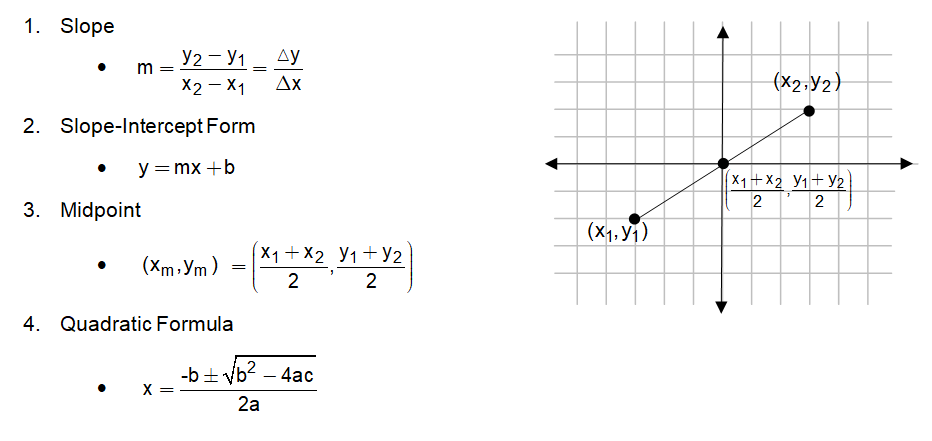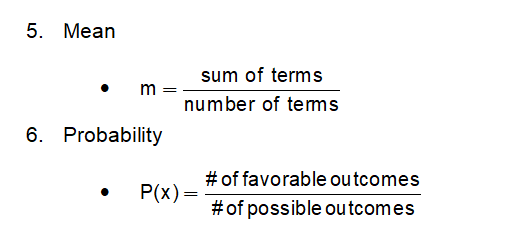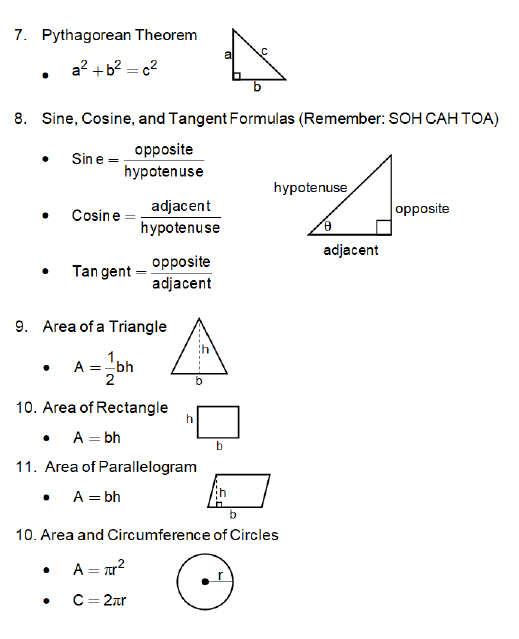# The Most Important Math Formulas to Know in High School

All High School mathematics students are familiar with learning and memorizing new formulas. There are hundreds of formulas, postulates, and theorems that they are expected to understand and implement on homework, quizzes, and tests. But should students memorize all of them? What are the most important math formulas to know?

# Important High School Math Formulas by Topic

## Algebra

The most important algebraic math formulas to know for are the ones for slope, slope-intercept form, midpoint, and the ever-famous quadratic formula. These four formulas are needed in each year of high school mathematics.## Statistics & Probability

Statistics and probability topics are covered in nearly every mathematics course. Students can also take them as separate courses in most high schools (showing the significance of the topic).  Furthermore, standardized tests love to include questions about them. That’s why knowing how to find the mean (average) and the likelihood of an event happening are crucial to success on these tests.## Geometry

Geometry is the topic containing the most math formulas. It’s a subject that makes some students feel overwhelmed by thanks to all of formulas, theorems, and postulates. If you are one of those students, don’t worry! Here are the most important formulas for Geometry.For high school students, these formulas are the most important math formulas to know. Students who understand and can put into practice these formulas are more likely to succeed in the classroom and on standardized testing, especially the ACT/SAT.

Want to know more about the formulas above? Ask us in the comments!

Author: Nate Baltzer, Writer and Teacher at A Grade Ahead

#### Get Notified When New Articles Post

Sign up to receive one email per week from A Grade Ahead full of parenting tips and helpful articles. Enter your information on the right hand side of our Blog page and we will add you to our list of subscribers. We also welcome your feedback and comments on our posts!

## Subscribe

Don't miss the latest article! Stay up-to-date on our blog posts by subscribing below.